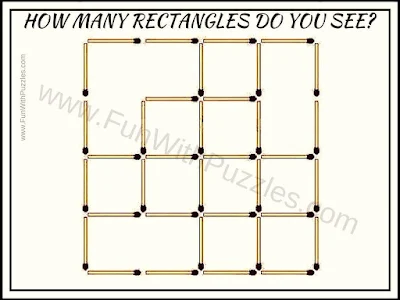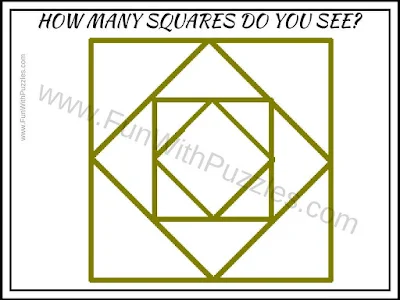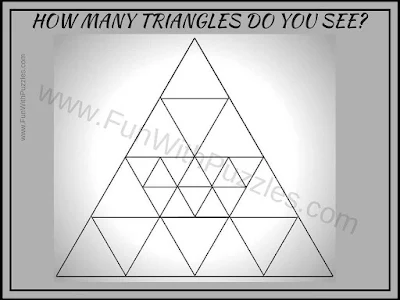Observational picture puzzles are one of the very interesting puzzle types. Observational puzzles include different types of visual puzzles and counting the shapes is one of the puzzle types which is a subset of Observational picture puzzles. Counting the number of squares in the given puzzle picture is a very famous puzzle on the Fun With Puzzles website. So here are similar picture puzzles in which one has to count the number of squares, triangles, or rectangles. Can you find the answers to each of these picture puzzles correctly on your first attempt?How Many Rectangles do you see in this picture?How Many Triangles do you see in this picture?How Many rectangles can you count in this picture?How many Squares can you count in this picture?How many triangles can you see in this picture?

Unknown said...

1